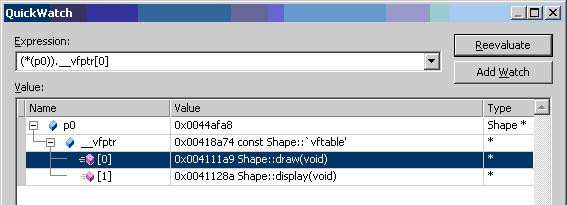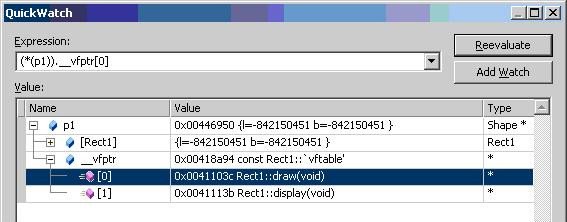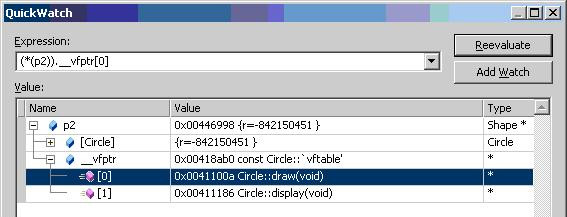# C++ - Virtual Functions

virtual functions are the functions can be overridden by the derived classes by changing its default behavior. I have explained how the concept of virtual function works in derived classes with example.

By including one or more virtual functions will increase the size of the class by 4 bytes. This 4 bytes are used to store the address of the array of function pointers. In the following sample code, we have got two virtual functions.

In the main function, we have got 4 Shape* objects p0, p1, p2 and p3 and all objects are calling its corresponding derived class draw function. Lets check the function pointer table on debugger for all the 4 objects. The following are the screenshots for all 4 objects.Even if p0, p1, p2 and p3 are the pointers to Shape object, their address pointing to array of virtual function table are different. From the screen shot given above, we can see that the different function addresses are used when we make a call to draw function from the base class pointer object.

0x004111a9 Shape::draw function
0x0041103c Rect1::draw function
0x0041100a Circle::draw function
0x00411293 Square::draw function

## Source Code

#include <iostream>

#include <tchar.h>

class Shape

{

public:

Shape()

{

std::cout << "Shape\n";

}

virtual void draw() { }

virtual void display() { }

};

class Rect1 : public Shape

{

int l,b;

public:

Rect1()

{

std::cout << "Rectangle\n\n";

}

virtual void draw() {}

virtual void display() { }

};

class Circle : public Shape

{

int r;

public:

Circle()

{

std::cout << "Circle\n\n";

}

virtual void draw() {}

virtual void display() { }

};

class Square : public Shape

{

int a;

public:

Square()

{

std::cout << "Square\n\n";

}

virtual void draw() {}

virtual void display() { }

};

int _tmain(int argc, _TCHAR* argv[])

{

Shape *p0 = new Shape();

Shape *p1 = static_cast<Shape*> (new Rect1());

Shape *p2 = static_cast<Shape*> (new Circle());

Shape *p3 = static_cast<Shape*> (new Square());

p0->draw(); // calls Shape::draw() function

p1->draw(); // calls Rect1::draw() function

p2->draw(); // calls Circle::draw() function

p3->draw(); // calls Square::draw() function

return 0;

}

Shape

Shape
Rectangle

Shape
Circle

Shape
Square